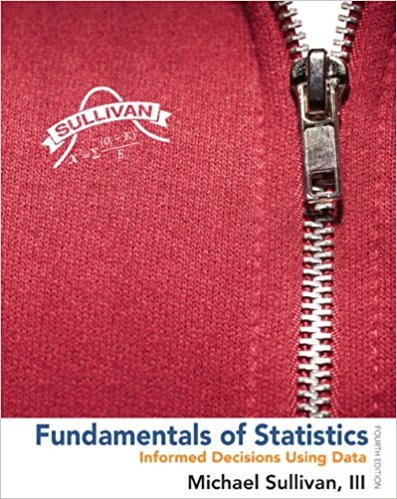×
×

# Solutions for Chapter Chapter 9.1: Fundamentals of Statistics 4th Edition## Full solutions for Fundamentals of Statistics | 4th Edition

ISBN: 9780321838704Solutions for Chapter Chapter 9.1

Solutions for Chapter Chapter 9.1
4 5 0 322 Reviews
27
2
##### ISBN: 9780321838704

This textbook survival guide was created for the textbook: Fundamentals of Statistics, edition: 4. This expansive textbook survival guide covers the following chapters and their solutions. Since 47 problems in chapter Chapter 9.1 have been answered, more than 306852 students have viewed full step-by-step solutions from this chapter. Chapter Chapter 9.1 includes 47 full step-by-step solutions. Fundamentals of Statistics was written by and is associated to the ISBN: 9780321838704.

Key Statistics Terms and definitions covered in this textbook
• 2 k factorial experiment.

A full factorial experiment with k factors and all factors tested at only two levels (settings) each.

• a-error (or a-risk)

In hypothesis testing, an error incurred by failing to reject a null hypothesis when it is actually false (also called a type II error).

• Alias

In a fractional factorial experiment when certain factor effects cannot be estimated uniquely, they are said to be aliased.

• Assignable cause

The portion of the variability in a set of observations that can be traced to speciic causes, such as operators, materials, or equipment. Also called a special cause.

• C chart

An attribute control chart that plots the total number of defects per unit in a subgroup. Similar to a defects-per-unit or U chart.

• Center line

A horizontal line on a control chart at the value that estimates the mean of the statistic plotted on the chart. See Control chart.

• Central composite design (CCD)

A second-order response surface design in k variables consisting of a two-level factorial, 2k axial runs, and one or more center points. The two-level factorial portion of a CCD can be a fractional factorial design when k is large. The CCD is the most widely used design for itting a second-order model.

• Completely randomized design (or experiment)

A type of experimental design in which the treatments or design factors are assigned to the experimental units in a random manner. In designed experiments, a completely randomized design results from running all of the treatment combinations in random order.

• Conditional mean

The mean of the conditional probability distribution of a random variable.

• Conidence coeficient

The probability 1?a associated with a conidence interval expressing the probability that the stated interval will contain the true parameter value.

• Continuous uniform random variable

A continuous random variable with range of a inite interval and a constant probability density function.

• Correlation

In the most general usage, a measure of the interdependence among data. The concept may include more than two variables. The term is most commonly used in a narrow sense to express the relationship between quantitative variables or ranks.

• Designed experiment

An experiment in which the tests are planned in advance and the plans usually incorporate statistical models. See Experiment

• Dispersion

The amount of variability exhibited by data

• Distribution function

Another name for a cumulative distribution function.

• Error propagation

An analysis of how the variance of the random variable that represents that output of a system depends on the variances of the inputs. A formula exists when the output is a linear function of the inputs and the formula is simpliied if the inputs are assumed to be independent.

• Estimate (or point estimate)

The numerical value of a point estimator.

• Exhaustive

A property of a collection of events that indicates that their union equals the sample space.

• Experiment

A series of tests in which changes are made to the system under study

• Factorial experiment

A type of experimental design in which every level of one factor is tested in combination with every level of another factor. In general, in a factorial experiment, all possible combinations of factor levels are tested.

×